# RD Sharma Solutions for Class 6 Chapter 7: Decimals Exercise 7.1

The word decimal comes from a Latin word ‘Decem’ which means 10. The basic concepts of decimals were discussed in earlier classes. RD Sharma Solutions Class 6 Exercise 7.1 is the first exercise which has problems based on decimals and writing the place value table for the given set of decimals. We at BYJU’S have a set of experts in the respective disciplines who prepare solutions which help students boost their exam preparation.RD Sharma Solutions for Class 6 Maths Chapter 7 Decimals Exercise 7.1 PDF are provided here.

## RD Sharma Solutions for Class 6 Chapter 7: Decimals Exercise 7.1 Download PDF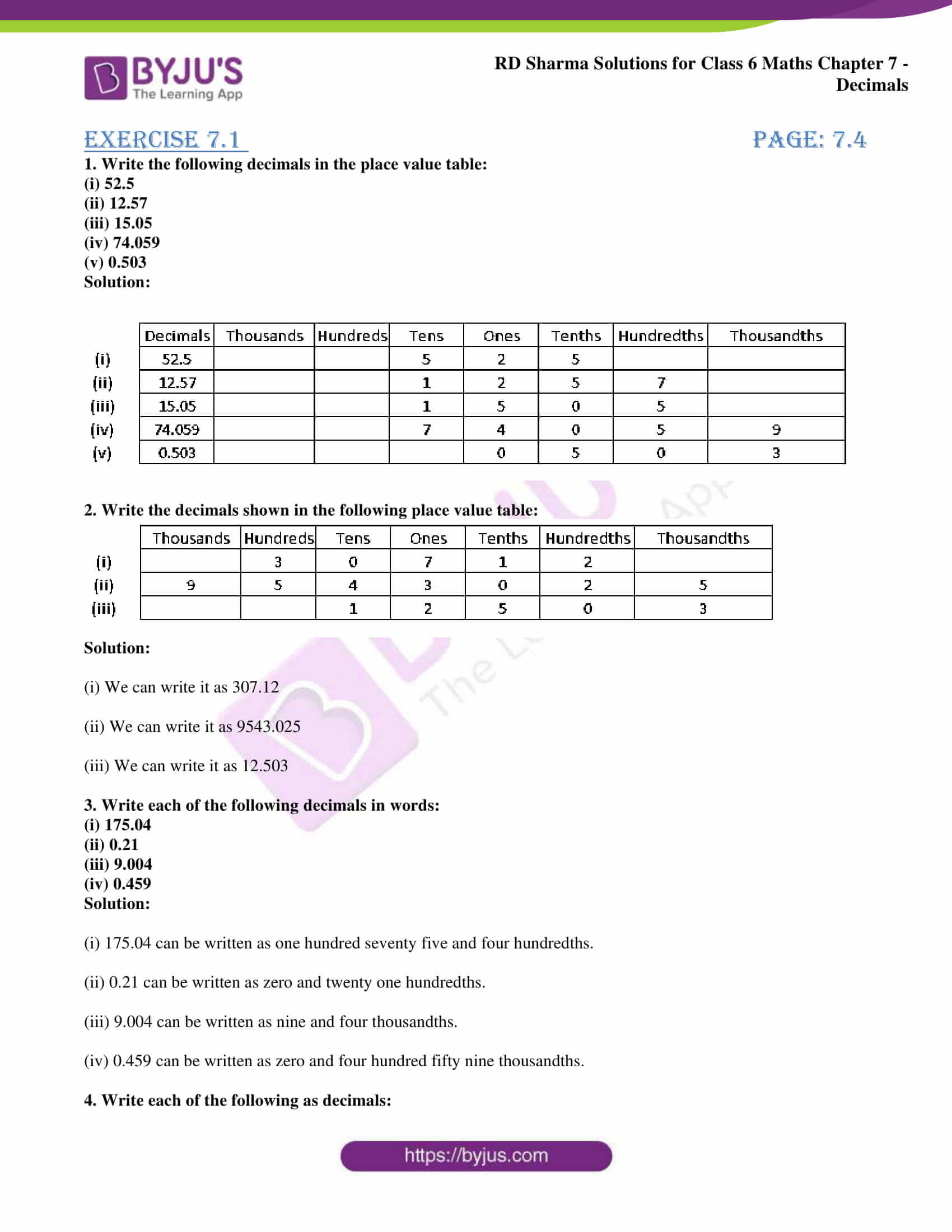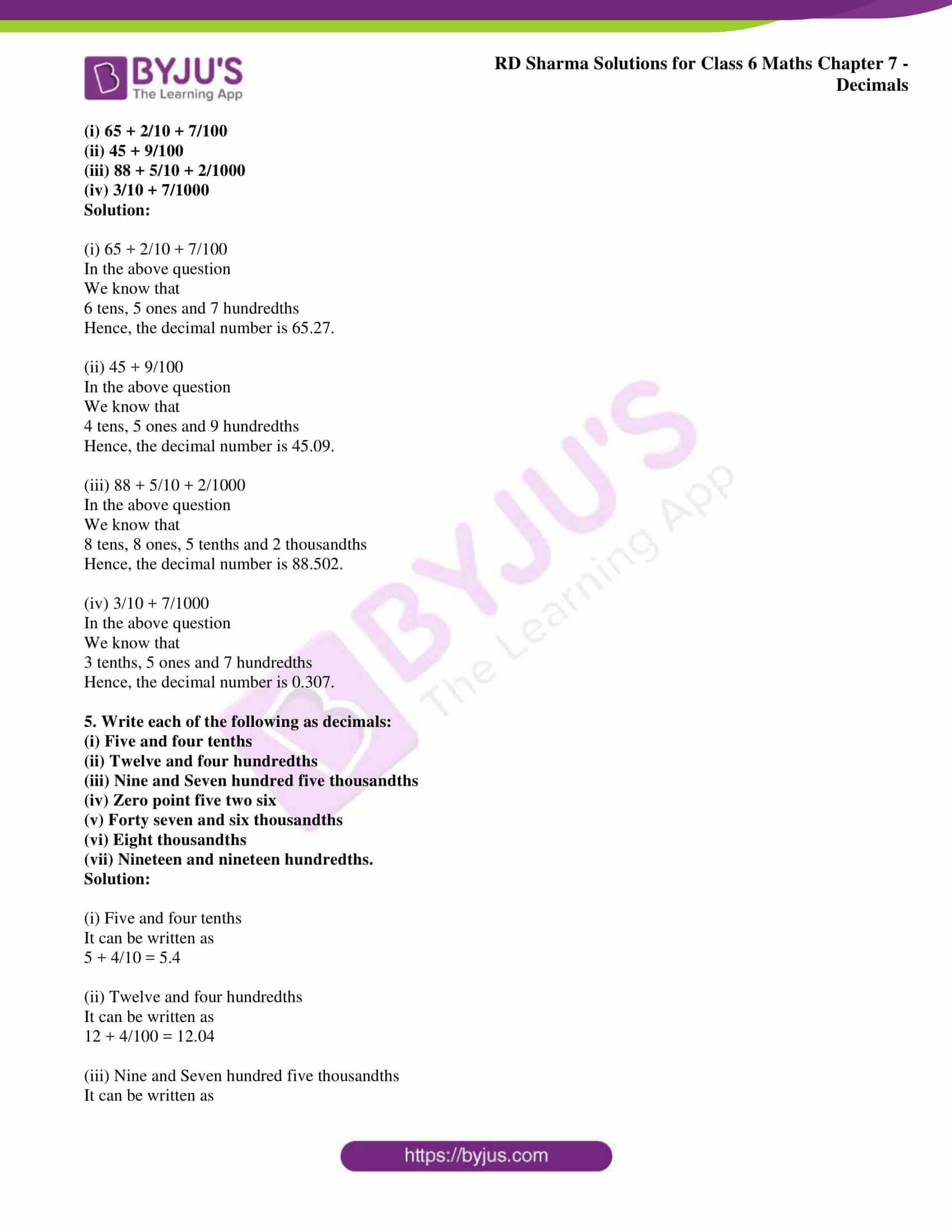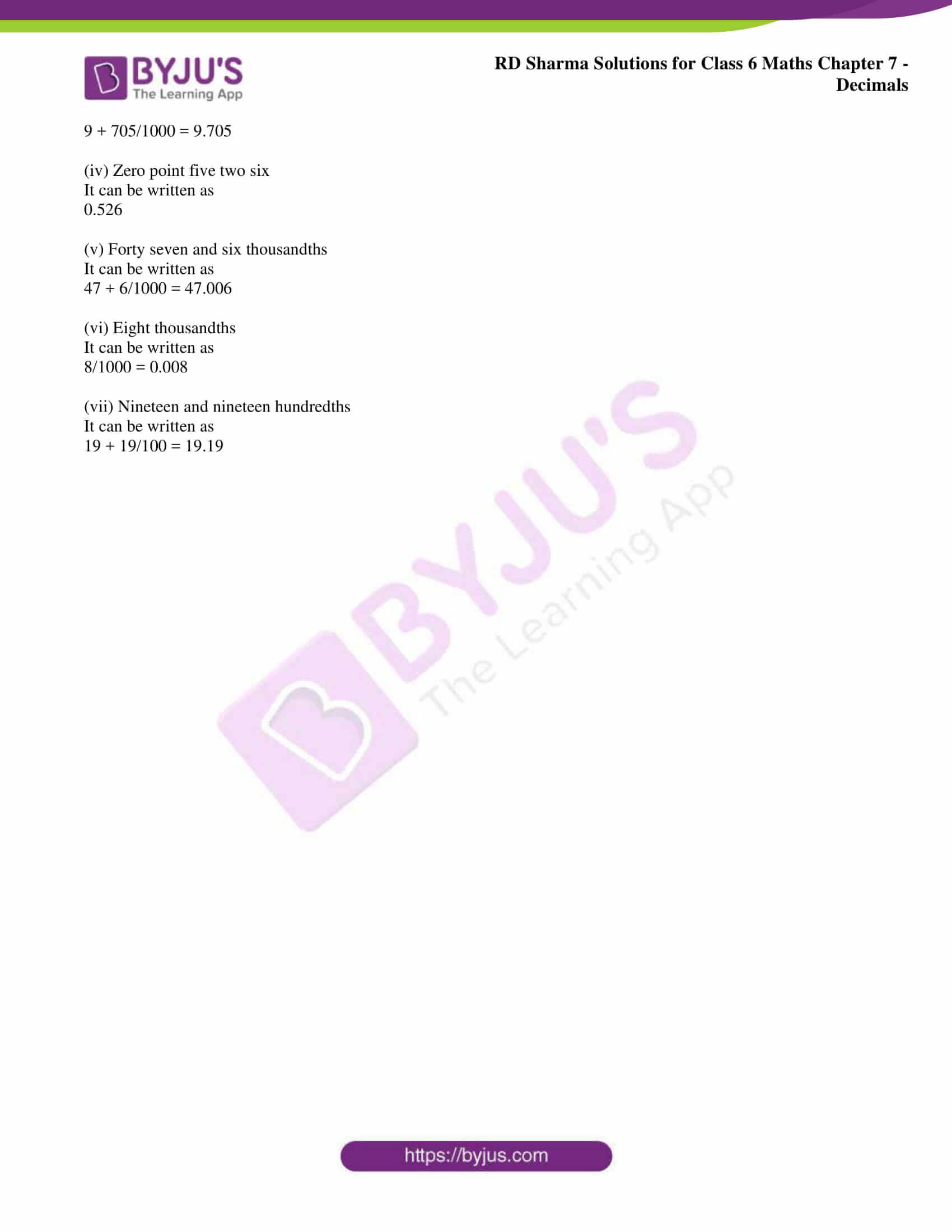### Access RD Sharma Solutions for Class 6 Chapter 7: Decimals Exercise 7.1

1. Write the following decimals in the place value table:

(i) 52.5

(ii) 12.57

(iii) 15.05

(iv) 74.059

(v) 0.503

Solution: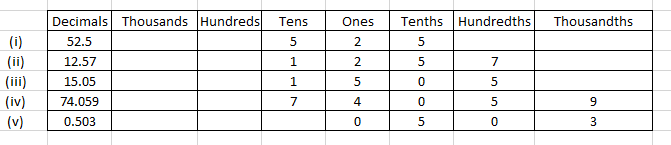2. Write the decimals shown in the following place value table: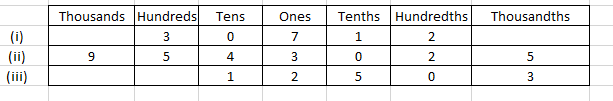Solution:

(i) We can write it as 307.12

(ii) We can write it as 9543.025

(iii) We can write it as 12.503

3. Write each of the following decimals in words:

(i) 175.04

(ii) 0.21

(iii) 9.004

(iv) 0.459

Solution:

(i) 175.04 can be written as one hundred seventy five and four hundredths.

(ii) 0.21 can be written as zero and twenty one hundredths.

(iii) 9.004 can be written as nine and four thousandths.

(iv) 0.459 can be written as zero and four hundred fifty nine thousandths.

4. Write each of the following as decimals:

(i) 65 + 2/10 + 7/100

(ii) 45 + 9/100

(iii) 88 + 5/10 + 2/1000

(iv) 3/10 + 7/1000

Solution:

(i) 65 + 2/10 + 7/100

In the above question

We know that

6 tens, 5 ones and 7 hundredths

Hence, the decimal number is 65.27.

(ii) 45 + 9/100

In the above question

We know that

4 tens, 5 ones and 9 hundredths

Hence, the decimal number is 45.09.

(iii) 88 + 5/10 + 2/1000

In the above question

We know that

8 tens, 8 ones, 5 tenths and 2 thousandths

Hence, the decimal number is 88.502.

(iv) 3/10 + 7/1000

In the above question

We know that

3 tenths, 5 ones and 7 hundredths

Hence, the decimal number is 0.307.

5. Write each of the following as decimals:

(i) Five and four tenths

(ii) Twelve and four hundredths

(iii) Nine and Seven hundred five thousandths

(iv) Zero point five two six

(v) Forty seven and six thousandths

(vi) Eight thousandths

(vii) Nineteen and nineteen hundredths.

Solution:

(i) Five and four tenths

It can be written as

5 + 4/10 = 5.4

(ii) Twelve and four hundredths

It can be written as

12 + 4/100 = 12.04

(iii) Nine and Seven hundred five thousandths

It can be written as

9 + 705/1000 = 9.705

(iv) Zero point five two six

It can be written as

0.526

(v) Forty seven and six thousandths

It can be written as

47 + 6/1000 = 47.006

(vi) Eight thousandths

It can be written as

8/1000 = 0.008

(vii) Nineteen and nineteen hundredths

It can be written as

19 + 19/100 = 19.19